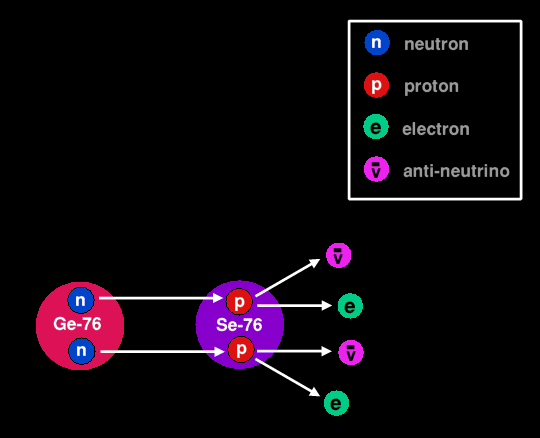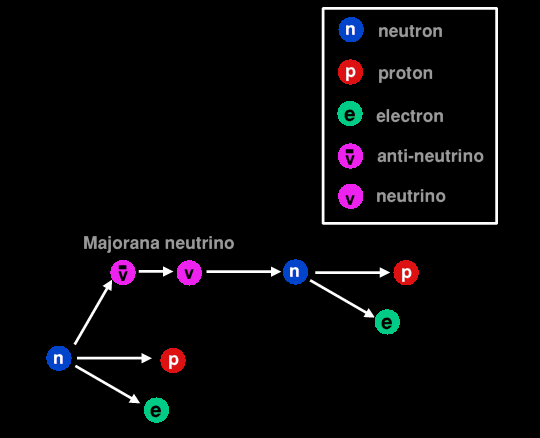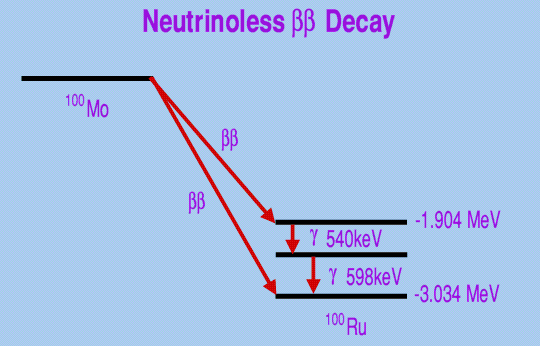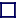### NEUTRINO-LESS DOUBLE BETA DECAYMAJORANA NEUTRINOS ??

There is a slight chance that a version of double-beta decay exists that involves the emission of no neutrinos. This so-called neutrino-less double beta decay should be impossible, but may not be so.

Normal double beta decay involves the almost simultaneous conversion of two neutrons into protons which in the process emit two electrons and two anti-neutrinos. See diagram below.Normal Double Beta Decay of Germanium-76
 Two neutrons in germanium-76 simultaneously decay into two protons plus two electrons and two anti-neutrinos transmuting it into selenium-76. This process conserves Lepton Number.

 Lepton Number and Lepton Number Conservation Leptons are light particles, electrons, and neutrinos, and so too are their anti-particles. All the lepton anti-particles have a lepton number of -1, whereas all the lepton particles have a lepton number of +1. All particles that are not leptons (baryons like the proton and neutron, for instance) have a lepton number of zero. In all observed reactions between particles the total Lepton Number is strictly conserved. But Lepton Number Conservation would be violated in neutrino-less double beta decay, if neutrino-less double beta decay can occur.

In 1937 the Italian physicist, Ettore Majorana, predicted that a form of double beta decay where no neutrinos are produced might be possible, but it was quickly pointed out that such a decay mode would not conserve lepton number, which seems to be a key requirement of physics. The electron has a lepton number of +1, and that of the anti-neutrino -1, and in normal double beta decay these two quantities cancel out (+2 -2 = 0), but in neutrino-less double beta decay the lepton number would increase by +2. [See box on Lepton Conservation]. No process has yet been found whereby the lepton number is not conserved. The process involved in neutrino-less double beta decay is a two-stage process: Firstly the isotope undergoes beta decay, where a neutron changes into a proton and emits an electron and an anti-neutrino. Secondly, a neutron in the intermediate isotope absorbs the anti-neutrino becoming a proton and emitting another electron, which is impossible (It would be possible for a neutron to absorb a neutrino and become a proton, but not with an anti-neutrino). For neutrino-less double beta decay to be possible at all, the emitted anti-neutrino must turn into a neutrino, which in effect means that anti-neutrinos and neutrinos are identical. This describes the Majorana neutrino. This situation would be unique: no other particle has an anti-particle that is identical to itself. The lepton number of all other leptons is +1, and -1 for all anti-leptons, so what would be the lepton number of the Majorana neutrino?Neutrino-less Double Beta Decay
 A neutron decays to a proton plus electron plus anti-neutrino, which changes into a neutrino before being absorbed by another neutron changing that into a proton and emitting another electron. No net neutrinos are emitted. Apart from that, neutrino-less double beta decay results in the same transmutation of one element to another as does normal double beta decay. This process, if it occurs, would violate Lepton Number Conservation.

But this 'impossibility' has not stopped experimentalists trying to see if ordinary double-beta decaying isotopes might, once in a while, decay by neutrino-less double beta decay. To this end, five cylinders of germanium (purified from the normal isotopic abundance of 8% Ge-76 to 80% Ge-76) [a double-beta decaying isotope] have been sited 1400 metres beneath an Italian mountain to shield it from cosmic rays and monitored since 1990 over a ten year period for neutrino-less double beta decay. The result was 30 suspected possible neutrino-less double beta decay events and a measured Majorana neutrino effective mass of 0.39eV. In double beta decay (and neutrino-less double beta decay) the germanium-76 transmutes into selenium-76 with the emission of two electrons. The electrons emitted by double beta decay events will have lower energies than any emitted by neutrino-less double beta decay events. With germanium being a semi-conductor, a measurable electrical current will be generated by both events, but the current will be slightly larger for any neutrino-less double beta decay events. If such neutrino-less double beta decay events turn out not to be measurement artifacts, then this result has wide-ranging implications. It would give clues to theorists on how to update the so-called standard model of sub-atomic particle physics. It would also give information on the mass of the lightest neutrino because the mass of that influences the reaction rate.

Speaking personally, I think that they would be better off using zinc-70, a double beta decaying isotope with an isotopic abundance of only 0.6% but with a very short half-life (for double beta decay) of just 500×1012years. It decays into germanium-70. The halflife of the germanium-76 which they are using is a massive 2 million times longer at 1.09×21years . It would, of course, boost the sensitivity still further by purifying the zinc-70 from 0.6% to above 50%. And if they really needed the material to be semi-conducting, they could use it as either the semi-conducting zinc telluride, or zinc selenide, using, of course, the double beta decaying tellurium-130 (isotopic abundance of 34.8%, halflife 790×1021)years) or the double beta decaying selenium-82 (isotopic abundance of 0.73%, halflife 121×1018. This would also give a double-check (which is missing from their original experimental procedure) on their measurements, since the double-beta decay energies are different for all three of these isotopes (1MeV for Zn-70, 2.52MeV for Te-130 and 2.99MeV for Se-82) [That for Ge-76 is 2.03MeV].

Other experiments using double beta decaying isotopes are either under construction (using Molybdenum-100) or proposed (using xenon-136, tellurium-130, calcium-40, neodymium-150, selenium-82 and cadmium-116).Energy-level chart of Neutrino-less Double Beta Decay of Mo-100 into Ru-100
 The measurement of the neutrino-less double beta decay of molybdenum-100 promises to be very accurate. If neutrino-less double beta decay is possible at all, there are two neutrino-less decay routes by which Mo-100 can decay into ruthenium-100; one by neutrino-less double-beta emission, and the other (although 40 times less probable) by the emission of two less energetic electrons to excited states of Ru-100, which then almost immediately decay to the ground state of Ru-100 by the emission of two gamma ray photons, one of 540KeV energy, the other of 598keV. It is this latter branch that will afford great immunity from background radiation, because by detecting these four almost-simultaneous particles with their corresponding energies background radiation can be almost eliminated. This is especially important for double beta decay experiments, where the probability of decay is minute because the halflife is so long (in this case 10.2×1018years, particularly when most double beta decays will be of the double-neutrino variety and therefore of less interest in any case.

If neutrino-less double beta decay is possible, and neutrinos are actually Majorana neutrinos, then this could help explain why matter dominates the Universe and there is no detectable anti-matter present. If Majorana neutrinos are the true nature of neutrinos, then a super-massive neutrino must also exist called a sterile neutrino, which would be expected to have a mass of as much as 1eV. [If they exist at all, a sterile neutrino, un-like the other neutrinos (electron neutrino, muon-neutrino and tau-neutrino) does not interact via the weak force, but only through gravity. Hence the name 'sterile']. In the immediate aftermath of the Big Bang, when the temperature of the Universe was extremely high, Majorana neutrinos would continually flip between being a super-massive neutrino and a Majorana neutrino. As the Universe cooled they would finally settle into their lighter partners, the Majorana neutrinos.